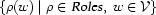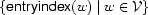### 5.4.7 Solving the CSP

We have now completely specified our constraint model. The CSP is obtained by collecting the constraints that its stipulates and then solving in terms of its variables: we need to look for assignments to the variables of the constraint model that satisfy its constraints. Here is the simple strategy that we follow:

• First, determine assignments to the daughter set variables. In other words, we apply a distribution strategy on:• Second, we make sure that a lexicon entry has really been selected for each word. I.e. we apply a distribution strategy on:This is sufficient to determine assignments to all variables.

Denys Duchier
Version 1.2.0 (20010221)